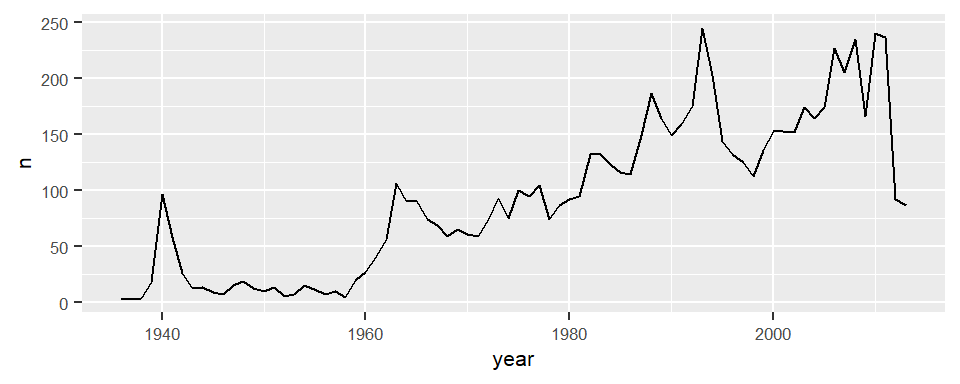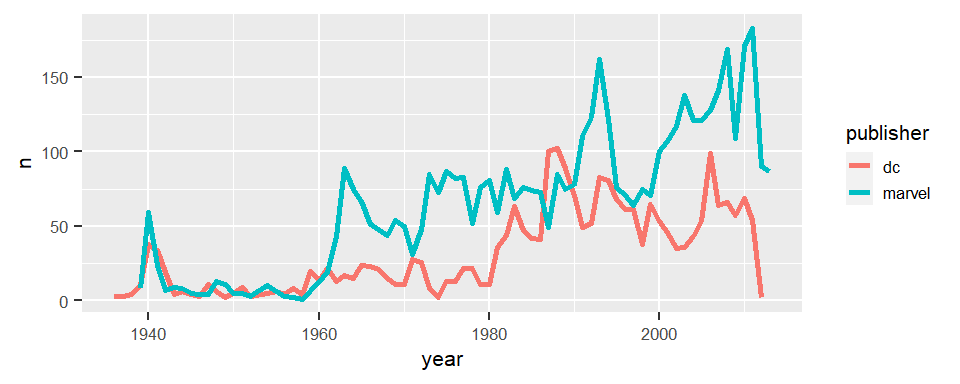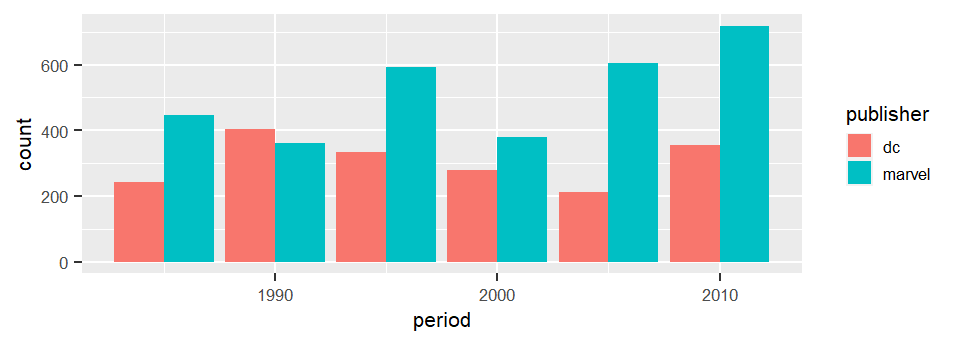🏠 Key Points :
■ Observe trends by line plot
■ Concatenate dplyr and ggplot in a pipeline
■ Comparing trends in various aspects at once

pacman::p_load(dplyr,tidyr,ggplot2,plotly,gridExtra)
theme_set(theme_get() + theme(
text=element_text(size=8), legend.key.size=unit(10,"points")
))
D = read.csv("data/comics1.csv") %>% as_tibble

### 1. Simple Line Plots

Let’s count and plot the numbers of new roles debuted annually.

count(D, year) %>%               # count the number of new roles per year
ggplot(aes(year, n)) + geom_line()  # and make a line plotcount() and ggplot() + geom_line() is a very handy combo. We can count by an extra group variable publisher to compare the trend lines of DC and Marvel.

count(D, year, publisher) %>%
ggplot(aes(year, n, col=publisher)) +
geom_line(size=1)##### 1.1 Debouncing the Choppy Trend Lines

The trend lines look pretty choppy. A common technique in observing long term trend is to increase the measurement period. Aggregation tends to cancel out random errors, thus smoooth the trend line. Since DC was not active before 1980, …

• filter out the data before 1980 and after 2010
• divide the 30 years into 6 periods
breaks=seq(1980,2010,5)
D2 = filter(D, year>=1980, year<=2010) %>%
mutate(period = cut(year,breaks,breaks[-1],T) %>%
as.character %>% as.integer)
table(D2\$period, useNA='ifany')

1985 1990 1995 2000 2005 2010
690  763  926  659  817 1073 
ggplot(D2, aes(period, fill=publisher)) +
geom_bar(position='dodge')It’s a lot easier to tell the long term trend, isn’t it. We see a valley at 2000 and Marvel seems to bounce back earlier and stronger than DC did.